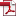## 페어링 암호 연산을 위한 $F_{3^m}$에서의 효율적인 세제곱근 연산 방법

Vol. 21, No. 2, pp. 3-12, 4월. 201110.13089/JKIISC.2011.21.2.3, Full Text:Keywords: Cube root, Shifted Polynomial Basis, finite field arithmetic
Abstract

$F_{3^m}$에서의 Tate 페어링 또는 ${\eta}_T$ 페어링 알고리즘 계산을 위하여 효율적인 세제곱근 계산은 매우 중요하다. $x^{1/3}$의 다항식 표현 중 0이 아닌 계수들의 개수를 $x^{1/3}$의 헤밍웨이트라 할 때, 이 헤밍웨이트가 세제곱근 연산의 효율성을 결정하게 된다. O. Ahmadi 등은 $f(x)=x^m+ax^k+b$ (a, $b{\in}F_3$)가 $F_3[x]$의 삼항 기약다항식이라 할 때, $F_{3^m}=F_3[x]/(f)$을 생성하는 모든 삼항 기약다항식에 대하여 $x^{1/3}$의 헤밍웨이트를 계산하였다. 본 논문에서는 Shifted Polynomial Basis(SPB)가 기존의 결과보다 $x^{1/3}$의 헤밍웨이트를 낮출 수 있음을 보이며, 모듈로 감산 연산이 필요 없는 가장 적합한 SPB를 제공한다.

Statistics
Y. Cho, N. Chang, C. Kim, Y. Park and S. Hong, "Efficient Formulas for Cube roots in $F_{3^m}$ for Pairing Cryptography," Journal of The Korea Institute of Information Security and Cryptology, vol. 21, no. 2, pp. 3-12, 2011. DOI: 10.13089/JKIISC.2011.21.2.3.
Young-In Cho, Nam-Su Chang, Chang-Han Kim, Young-Ho Park, and Seok-Hie Hong. 2011. Efficient Formulas for Cube roots in $F_{3^m}$ for Pairing Cryptography. Journal of The Korea Institute of Information Security and Cryptology, 21, 2, (2011), 3-12. DOI: 10.13089/JKIISC.2011.21.2.3.